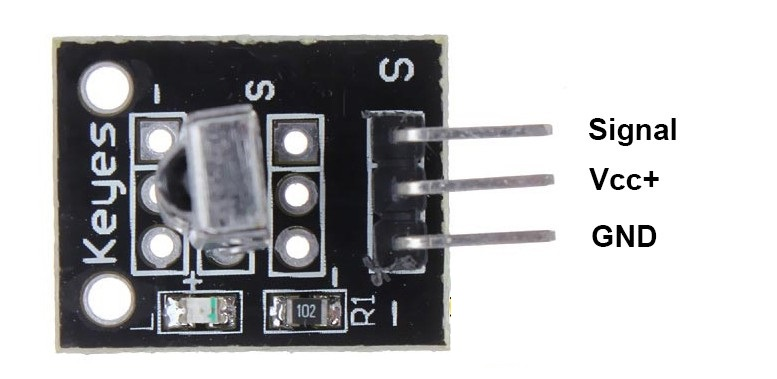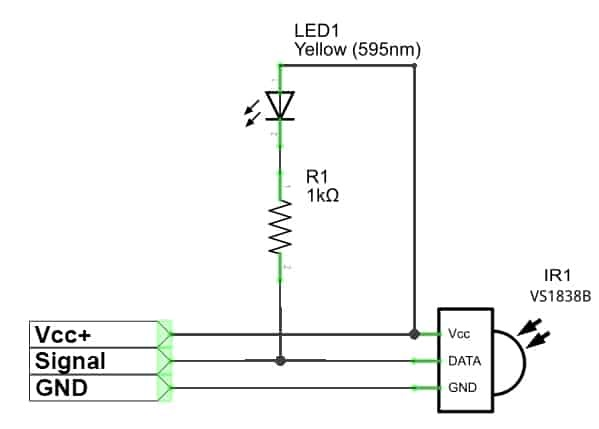# KY-022 INFRARED SENSOR Module

The KY-022 is equipped with three pins. It responds to a frequency of 38kHz at 940nm. This signal is sent via the digital output. If an IR signal is detected, the LED module on board will light up. This sensor module can be used to decode remote controls for home theaters and other remote controls that use IR.

Device Pinout and  Schematics:Package Content:

1 x KY-022 INFRARED SENSOR Module

This module has three pins:

• Signal
• Vcc+
• Ground.

## KY-022 INFRARED SENSOR Module for Arduino

Wiring with Arduino board:

• KY-022 Sensor GND - Arduino GND
• KY-022 Sensor Vcc+ - Arduino +5V
• KY-022 Sensor Signal - Arduino PIN 10## KY-022 INFRARED SENSOR Module connections with raspberry pi

```import RPi.GPIO as GPIO
from time import time

def setup():
GPIO.setmode(GPIO.BOARD) # Numbers GPIOs by physical location
GPIO.setup(11, GPIO.IN, pull_up_down=GPIO.PUD_DOWN)

def binary_aquire(pin, duration):
# aquires data as quickly as possible
t0 = time()
results = []
while (time() - t0) < duration:
results.append(GPIO.input(pin))
return results

# when edge detect is called (which requires less CPU than constant
# data acquisition), we acquire data as quickly as possible
data = binary_aquire(pinNo, bouncetime/1000.0)
if len(data) < bouncetime:
return
rate = len(data) / (bouncetime / 1000.0)
pulses = []
i_break = 0
# detect run lengths using the acquisition rate to turn the times in to microseconds
for i in range(1, len(data)):
if (data[i] != data[i-1]) or (i == len(data)-1):
pulses.append((data[i-1], int((i-i_break)/rate*1e6)))
i_break = i
# decode ( < 1 ms "1" pulse is a 1, > 1 ms "1" pulse is a 1, longer than 2 ms pulse is something else)
# does not decode channel, which may be a piece of the information after the long 1 pulse in the middle
outbin = ""
for val, us in pulses:
if val != 1:
continue
if outbin and us > 2000:
break
elif us < 1000:
outbin += "0"
elif 1000 < us < 2000:
outbin += "1"
try:
return int(outbin, 2)
except ValueError:
# probably an empty code
return None

def destroy():
GPIO.cleanup()

if __name__ == "__main__":
setup()
try:
print("Starting IR Listener")
while True:
print("Waiting for signal")
GPIO.wait_for_edge(11, GPIO.FALLING)
if code:
print(str(hex(code)))
else:
print("Invalid code")
except KeyboardInterrupt:
pass
except RuntimeError:
# this gets thrown when control C gets pressed
# because wait_for_edge doesn't properly pass this on
pass
print("Quitting")
destroy()
```Technical Current consumption 1.5mA peak Frequency 38kHz Operating Voltage 3.3V to 5V Pulse Duration 400µs to 800µs Range 17m Wavelength 940 nm

Be the first to ask a question.

#### Write a review

Note: HTML is not translated!